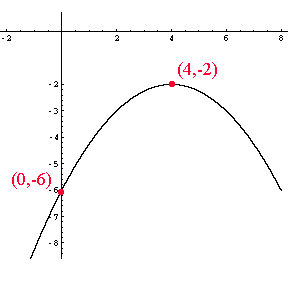Quandaries and Queries 12 grade, algebra II Given the vertex (4, -2) y intercept = -6 find if/where the parabola crosses the x axis? I'm subbing for a math class, and just can't remember. Thanks for any help you can offer. Hi Delores, First plot the two points (4,-2) and (0,-6) on the plane and then draw a rough sketch of what the parabola.This is a parabola that opens downward. I know that the "standard" parabola with vertex at (0,0) and which opens downward has equation y = - x2 The parabola you want is shifted 4 units to the right so the "x" factor should be (x - 4). The graph is then shifted up or down (in this case down) and then scaled (either expanded or contracted) to give the parabola you want. Hence I know that the equation is y = - a(x - 4)2 + b Now you can determine a and b since the parabola passes through (4,-2) and (0,-6). -2 = - a(4 - 4)2 + b = b so b = -2, and -6 = - a(-4)2 - 2 so a = 1/4. I don't know what detail you require to actually answer the question. It may be that the sketch at the beginning is enough to see that the parabola does not cross the x-axis. Penny Go to Math Central